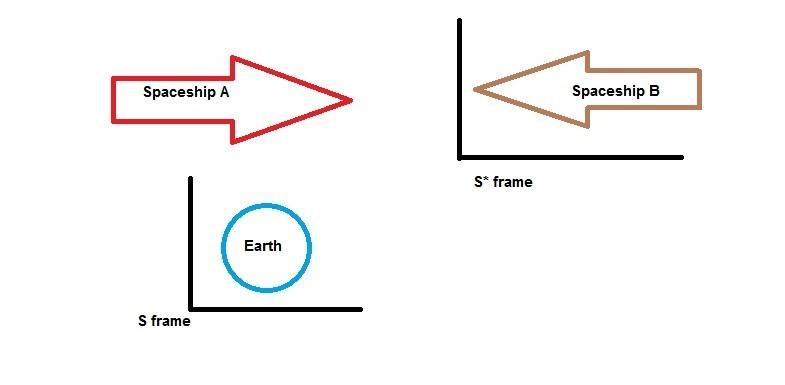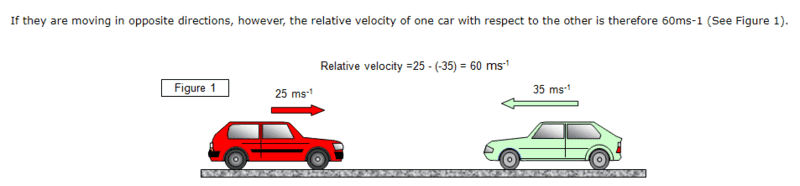## Homework Statement

Two spaceships pass each other. Spaceship A moves relative to nearby planet at velocity v1 , while spaceship B moves at velocity v2 relative to the planet . How fast does spaceship A moves relative to spaceship B.

## Homework Equations

Velocity Transformation : v* = (v-u)/(1-(vu/c2))

Inverse Velocity Transformation : v= (v*+u)/(1+(v*u/c2))

## The Attempt at a Solution

Let planet be the S frame
Let spaceship B be the S* frame
Let spaceship A be the event
Then,

v= velocity of event according to S frame ( here planet)
v* = velocity of the event according to S* frame (Here spaceship B)
u= relative velocity of S* frame wrt to S frame
event = Spaceship A

Using these :
v= v1
u=-v2
v* = V (which we have to find)

Using the inverse velocity transformation I get the solution :

V= v1+v2/(1+(v1v2/c2))

But correct solution given in the text book is

V = |v1-v2|/(1-(v1v2/c2)

Can some one help me with this ! :)

Ray Vickson
Homework Helper
Dearly Missed

## Homework Statement

Two spaceships pass each other. Spaceship A moves relative to nearby planet at velocity v1 , while spaceship B moves at velocity v2 relative to the planet . How fast does spaceship A moves relative to spaceship B.

## Homework Equations

Velocity Transformation : v* = (v-u)/(1-(vu/c2))

Inverse Velocity Transformation : v= (v*+u)/(1+(v*u/c2))

## The Attempt at a Solution

Let planet be the S frame
Let spaceship B be the S* frame
Let spaceship A be the event
Then,

v= velocity of event according to S frame ( here planet)
v* = velocity of the event according to S* frame (Here spaceship B)
u= relative velocity of S* frame wrt to S frame
event = Spaceship A

Using these :
v= v1
u=-v2
v* = V (which we have to find)

Using the inverse velocity transformation I get the solution :

V= v1+v2/(1+(v1v2/c2))

But correct solution given in the text book is

V = |v1-v2|/(1-(v1v2/c2)

Can some one help me with this ! :)

You wrote
$$V = v_1 + \frac{v_2}{1 + v_1 v_2/c^2},$$
whiich is wrong. Perhaps you meant
$$V = \frac{v_1 + v_2}{1+ v_1 v_2/c^2}.$$
If so, you must use parentheses, like this: ## V = (v_1 + v_2)/(1+v_1 v_2/c^2)##, because an expression of the form a +b/c means ##a + \frac{b}{c}##, but (a+b)/c means exactly what it says.

Anyway, even after fixing that, you still have a problem with having ##v_1+v_2## instead of ##v_1-v_2##. Can you see why?

Yes sorry , that was a typo. I meant (v1+v2)/(1+(v1v2/c2))

No ! I don't have clue , what is wrong in my calculation :(

Apart from that question , which tool you are using to write equations in an interactive manner?

PeroK
Homework Helper
Gold Member
2020 Award
No ! I don't have clue , what is wrong in my calculation :(

What would be the answer in Newtonian physics? Try that first.

PeroK
Homework Helper
Gold Member
2020 Award
Apart from that question , which tool you are using to write equations in an interactive manner?

Latex. There's a guide here:

https://www.physicsforums.com/help/latexhelp/

If you "reply" to Ray's post above that will give you the latex to cut and paste to your post. To get you started.

•Hamza Abbasi
What would be the answer in Newtonian physics? Try that first.
I guess that would be v1+v2 . Right?

PeroK
Homework Helper
Gold Member
2020 Award
I guess that would be v1+v2 . Right?

It's a poor question, I think. It gives velocities ##v_1, v_2## but it asks "how fast", by which it means the relative speed.

In any case, the required answer is a speed, not a velocity.

•Hamza Abbasi
It's a poor question, I think. It gives velocities ##v_1, v_2## but it asks "how fast", by which it means the relative speed.

In any case, the required answer is a speed, not a velocity.
My solution for velocity is correct then?

PeroK
Homework Helper
Gold Member
2020 Award
My solution for velocity is correct then?

You're not thinking the problem through. First, you need to pick the reference frame of A or B. Then, you need to think about the velocities in this frame. Then you apply velocity addition. To get you started:

In the reference frame of B the velocity of the planet is ##-v_2##

You're not thinking the problem through. First, you need to pick the reference frame of A or B. Then, you need to think about the velocities in this frame. Then you apply velocity addition. To get you started:

In the reference frame of B the velocity of the planet is ##-v_2##
Sorry I don't get it.
In the reference frame of B , B would be stationary . Both the planet and spaceship would be approaching it from positive X direction. This makes the velocity of the planet to be +v2 . And the relative velocity in B's frame of reference in Newtonian physics will be , v1+v2.

This is what I am imaging#### Attachments

PeroK
Homework Helper
Gold Member
2020 Award
Sorry I don't get it.
In the reference frame of B , B would be stationary . Both the planet and spaceship would be approaching it from positive X direction. This makes the velocity of the planet to be +v2 . And the relative velocity in B's frame of reference in Newtonian physics will be , v1+v2.

In your diagram you have spaceship B going in the opposite direction. If, say, we have ##v_A = +2m/s## and ##v_B = +1m/s##, then the relative (Newtonian) velocity (of A in B's frame) is ##v_A' = v_A - v_B = +1m/s##.

You are treating the velocities of A and B as speeds in opposite directions.

In your diagram you have spaceship B going in the opposite direction. If, say, we have ##v_A = +2m/s## and ##v_B = +1m/s##, then the relative (Newtonian) velocity (of A in B's frame) is ##v_A' = v_A - v_B = +1m/s##.

You are treating the velocities of A and B as speeds in opposite directions.
Sorry I don't understand why we are subtracting the velocities? Don't they add up when the two bodies are moving in opposite direction relative to each other?#### Attachments

PeroK
Homework Helper
Gold Member
2020 Award
Sorry I don't understand why we are subtracting the velocities? Don't they add up when the two bodies are moving in opposite direction relative to each other?View attachment 224607

That is subtraction. It's ##25m/s - (-35m/s) = 60 m/s##.

I think you are confused by speeds and velocities. In this case, you subtract the negative velocity, which is the same as adding the speeds.

PS If you given general velocities, like ##v_1, v_2## you really need to have them pointing in the same direction in any diagram. If you are given specific velocities, like ##+25m/s, -35m/s## then you can show them in opposite directions.

Ray Vickson
Homework Helper
Dearly Missed
Sorry I don't understand why we are subtracting the velocities? Do n't they add up when the two bodies are moving in opposite direction relative to each other?View attachment 224607

Let's look at the simpler case of classical velocity addition. Suppose I am driving in car 1 at velocity v1 and you are driving in car 2 at velocity v2. If v1 = 60 km/hr and v2 = 60 km/hr, we are driving side-by-side at the same speed, so our relative velocity is 0, and v1 - v2 = 0. Your formula would give v_relative = v1 + v2 = 120 km/h, so you would have us speeding rapidly apart. Now suppose we are driving in opposite directions, so v1 = + 60 and v2 = -60. In a head-on-collision our two cars would come together at a speed of 120 km/hr, leaving them as two crumbled masses of metal. Note that v_relative = v1 - v2 = 60 - (-60) = 120. However, YOUR calculation would give a relative velocity of v1 + v2 = 0, so the cars would not suffer any damage at all---not even scratched paint jobs.

The point is that velocity is a vector quantity, having both magnitude and direction; the subtraction formula v_relative = v1 - v2 takes care of all the possibilities.

•Hamza Abbasi and PeroK
That is subtraction. It's ##25m/s - (-35m/s) = 60 m/s##.

I think you are confused by speeds and velocities. In this case, you subtract the negative velocity, which is the same as adding the speeds.

PS If you given general velocities, like ##v_1, v_2## you really need to have them pointing in the same direction in any diagram. If you are given specific velocities, like ##+25m/s, -35m/s## then you can show them in opposite directions.
Okay !!
I think I get it.
The velocity v2 already include negative sign and magnitude in it ? I don't need to put an extra negative sign in it ,Right?

PeroK
Homework Helper
Gold Member
2020 Award
Okay !!
I think I get it.
The velocity v2 already include negative sign and magnitude in it ? I don't need to put an extra negative sign in it ,Right?

##v_2## is unspecified. You might infer from the problem that ##v_1 > 0## and ##v_2 < 0##, but it doesn't actually say that. It doesn't even say they are travelling in opposite directions. You could assume that ##v_1 > 0## and ##v_2 < 0##, but the forumula for relative velocity is still ##v_1 - v_2##.

To do what you want to do, you would have to assume that ##v_1 > 0## and ##v_2 = -u##, where ##u > 0##. Then, the velocity of A relative to B would be ##v_1 - v_2 = v_1 + u##.

But, really you should be thinking that in general ##v_1, v_2## can both be positive or negative.

In fact, even if you take ##v_1 = v_2##, then the answer given in the book is still correct. It's irrelevant, in other words, whether they ever pass by each other. That condition could, in fact, be omitted from the question.

•Hamza Abbasi
##v_2## is unspecified. You might infer from the problem that ##v_1 > 0## and ##v_2 < 0##, but it doesn't actually say that. It doesn't even sayu they are travelling in opposite directions. You could assume that ##v_1 > 0## and ##v_2 < 0##, but the forumula for relative velocity is still ##v_1 - v_2##.

To do what you want to do, you would have to assume that ##v_1 > 0## and ##v_2 = -u##, where ##u > 0##. Then, the velocity of A relative to B would be ##v_1 - v_2 = v_1 + u##.

But, really you should be thinking that in general ##v_1, v_2## can both be positive or negative.

In fact, even if you take ##v_1 = v_2##, then the answer given in the book is still correct. It's irrelevant, in other words, whether they ever pass by each other. That condition could, in fact, be omitted from the question.
Thank you I get in it now !
You are very patient , thank you for tolerating me !Good Luck

•PeroK